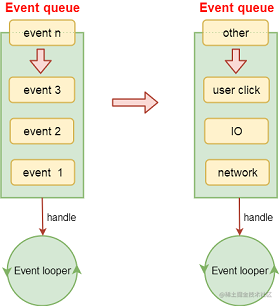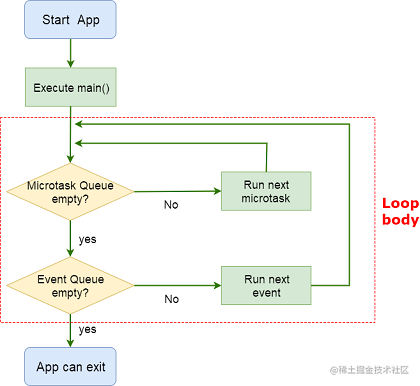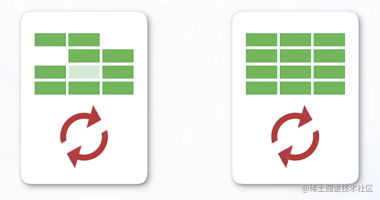# Flutter - Dart事件循环机制与异步

## 一、Dart 异步

### 1、同步与异步

• 同步: 同一线程中, 按照代码的编写顺序, 自上而下依次执行 (直观感受: 需要等待)
• 异步: 代码执行中, 某段代码的执行不会影响后面代码的执行 (直观感受: 无需等待)

### 2、单线程模型

#### 单线程模型:

• 一条执行线上, 同时且只能执行一个任务(事件), 其他任务都必须在后面排队等待被执行.
• 为了不阻碍代码的执行, 每遇到的耗时任务都会被挂起放入任务队列, 待执行结束后再按放入顺序依次执行队列上的任务, 从而达到异步效果.

#### 单线程模型 与 多线程 各自的优势:

• 单线程模型的优势: 避免了多线程的缺点, 比较适合于需要等待对方传送数据或返回结果的耗时操作, 如网络请求, IO 操作等.
• 多线程的优势: 尽可能利用处理器的多核实现并行计算的计算密集型操作.

#### 多线程 的缺点:

• 会带来额外的资源和性能消耗.
• 多个线程操作共享内存时需要加锁控制, 锁竞争会降低性能和效率, 复杂情况下还容易造成死锁.

### 3、事件循环机制Dart 事件循环机制由 一个`消息循环(Event Looper)` 和 两个`消息队列(Event Queue)` 构成, 这两个消息队列分别是: `事件队列(Event queue)``微任务队列(MicroTask queue)`.

#### Event Looper

Dart 在执行完 main 函数后, `Event Looper` 就开始工作, `Event Looper` 优先全部执行完 `Microtask Queue` 中的 event, 直到 `Microtask Queue` 为空时, 才会执行 `Event Looper` 中的 event, `Event Looper` 为空时才可以退出循环.#### Event Queue

`Event Queue` 的 event 来源于 `外部事件``Future`

• 外部事件: 例如输入/输出, 手势, 绘制, 计时器, Stream 等
• Future: 用于自定义 Event Queue 事件

``````Future(() {
// 事件任务
});

• `Microtask Queue` 的优先级高于 `Event Queue`.

Microtask 一般用于非常短的内部异步动作, 并且任务量非常少, 如果微任务非常多, 就会造成 Event Queue 排不上队, 会阻塞 Event Queue 的执行(如: 用户点击没有反应). 所以, 大多数情况下优先考虑使用 Event Queue, 整个 Flutter 源代码仅引用 `scheduleMicroTask()` 方法 7 次.

``````scheduleMicrotask(() {
// 微任务
});

## 二、Future

Dart 中的异步操作主要使用 `Future``async/await`, 整体与前端 ES6 中的 `Promise`, `async/await` 的使用差不多, 可以把 `Future` 理解为是一个自带 callback 效果的类.

### 1、基本使用

``````factory Future(FutureOr<T> computation()) {
...
}

``````Future<String> getNetworkData() {
// 1. 将耗时操作包裹到Future的回调函数中
return Future<String>(() {
sleep(Duration(seconds: 2));

return "Hello lqr"; // 只要有返回结果, 那么就执行Future对应的then的回调(相当于Promise-resolve)
// throw Exception("error"); // 如果没有结果返回(有错误信息), 需要在Future回调中抛出一个异常(相当于Promise-reject)
});
}

Future 实例有 3 个常用方法:

• then((value){...}): 正常运行时执行
• catchError((err){...}): 出现错误时执行
• whenComplete((){...}): 不管成功与否都会执行

``````main(List<String> args) {
print("main start");

// 2. 拿到结果
var future = getNetworkData();
future.then((value) => print(value)) // Hello lqr
.catchError((err) => print(err))
.whenComplete(() => print("执行完成")); // 不管成功与否都会执行

print("main end");
}

``````main start
main end

// 2秒后输出:
Hello lqr

``````var future = getNetworkData();

// 错误写法:
future.then((value) => print(value));
future.catchError((error) => print(error)); // 无效

// 正确写法:
future = future.then((value) {
print(value);
return value;
});
future.catchError((error) => print(error)); // 有效

### 2、链式调用

Future 可以在 then()方法中返回另一个 Future 实例, 从而达到链式调用的效果, 这对那些有数据关联的网络请求很有用:

``````main(List<String> args) {
print("main start");

// 链式调用, 执行多个数据处理
Future(() {
return "第一次结果";
}).then((value) {
print(value);
return "第二次结果";
}).then((value) {
print(value);
return "第三次结果";
}).then((value) {
print(value);
}).catchError((error) {
print(error);
});

print("main end");
}

### 3、其它 API

Future 除了默认构造器外, 还提供了几个常用的命名构造器:

• Future.value(): 创建一个返回具体数据的 Future 实例
• Future.error(): 创建一个返回错误的 Future 实例
• Future.delayed(): 创建一个延时执行的 Future 实例
``````main(List<String> args) {
print("main start");

Future.value("Hello lqr").then((value) => print(value));

Future.error("出错了").catchError((error) => print(error));

Future.delayed(Duration(seconds: 3))
.then((value) => "Hello lqr")
.then((value) => print(value));

Future.delayed(Duration(seconds: 2), () => "Hello lqr")
.then((value) => print("welcome"))
.then((value) => throw Exception("出错了"))
.catchError((error) => print(error))
.whenComplete(() => print("执行完成")); // 不管成功与否都会执行

print("main end");
}

## 三、async/await

`async/await` 是 Dart 提供的可以用 `同步的代码格式` 实现 `异步的调用过程``语法糖`.

### 1、基本使用

• `await` 必须在 `async` 函数中使用
• `async` 函数返回的结果必须是一个 Future
``````Future getNetworkData() async {
var userId = await getUserId();
var userInfo = await getUserInfo(userId);
}

``````Future getNetworkData() {
return getUserId().then((userId) {
return getUserInfo(userId);
}).then((userInfo) {
});
}

## 四、isolate### 1、创建 isolate (Dart API)

Dart 默认提供了 `Isolate.spawn(entryPoint, message)` 用于开启 `isolate`, 通过源码可以知道形参 `message` 其实是 形参 `entryPoint` 对应的函数执行时需要的参数:

``````external static Future<Isolate> spawn<T>(
void entryPoint(T message), T message,
{bool paused = false,
bool errorsAreFatal = true,
SendPort? onExit,
SendPort? onError,
@Since("2.3") String? debugName});

``````import 'dart:isolate';

main(List<String> args) {
print("main start");

Isolate.spawn(calc, 100);

print("main end");
}

void calc(int count) {
var total = 0;
for (var i = 0; i < count; i++) {
total += i;
}
print(total);
}

### 2、isolate 通信 (单向)

`isolate` 间可以一起工作的唯一方法是通过来回传递消息. 一般情况下, `子isolate` 会将运行结果通过管道以消息的形式发送到 `主isolate`, 并在 `主isolate``Event Looper` 中处理该消息, 这时就需要借助 `ReceivePort` 来处理消息的传递了:

• 在启动 `子isolate` 时, 将 `主isolate` 的发送管道(`SendPort`)作为参数传递给 `子isolate`.
• `子isolate` 在执行完毕时, 可以利用管道(`SendPort`)给 `主isolate` 发送信息.
``````import 'dart:isolate';

main(List<String> args) async {
print("main start");

// 1. 创建管道

// 2. 创建isolate
Isolate isolate = await Isolate.spawn(foo, receivePort.sendPort);

// 3. 监听管道
print(message);
// 不再使用时, 关闭管道
// 不再使用时, 将 isolate 杀死
isolate.kill();
});

print("main end");
}

void foo(SendPort sendPort) {
sendPort.send("Hello lqr");
}

### 3、创建 isolate (Flutter API)

Flutter 提供了更为方便的开启 `isolate` 的 API: `compute()` 函数. 以下是示例代码:

``````main(List<String> args) async {
int result = await compute(powerNum, 5);
print(result);
}

int powerNum(int num) {
return num * num;
}

`compute()` 是 Flutter 的 API, 不是 Dart 的 API, 所以, 上面的代码只能在 Flutter 项目中才能运行.